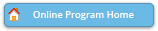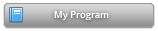#### Abstract Details

 Activity Number: 547 - Annals of Statistics Special Invited Session: Selected Papers Type: Invited Date/Time: Wednesday, July 31, 2019 : 2:00 PM to 3:50 PM Sponsor: IMS Abstract #300090 Title: Convergence Rates of Least Squares Regression Estimators with Heavy-Tailed Errors Author(s): Qiyang Han and Jon A. Wellner* Companies: Rutgers University and University of Washington Keywords: least squares; nonparametric; entropic dimension; heavy tailed errors; multiplier inequality Abstract: We study the performance of the Least Squares Estimator (LSE) in a general nonparametric regression model, when the errors are independent of the covariates but may only have a p-th moment with p larger than 1. In such a heavy-tailed regression setting, we show that if the model satisfies a standard entropy condition' with exponent $\alpha \in (0,2)$, then the squared error loss of the LSE converges at a rate given by the larger of the usual rate with Gaussian errors, $n^{-1/(2+\alpha)}$ and the rate $n^{-1/2 + 1/(2p)$ determined by the errors. This rate quantifies both positive and negative aspects of the LSE in a heavy-tailed regression setting. The validity of the above rate relies crucially on the independence of the covariates and the errors. In fact, the $L_2$ loss of the LSE can converge arbitrarily slowly when the independence fails. The key technical ingredient is a new multiplier inequality that gives sharp bounds for the multiplier empirical process' associated with the LSE. We further give an application to the sparse linear regression model with heavy-tailed covariates and errors to demonstrate the scope of this new inequality.

Authors who are presenting talks have a * after their name.